Vector product - Defination

Chapter 10 Class 12 Vector Algebra
Concept wise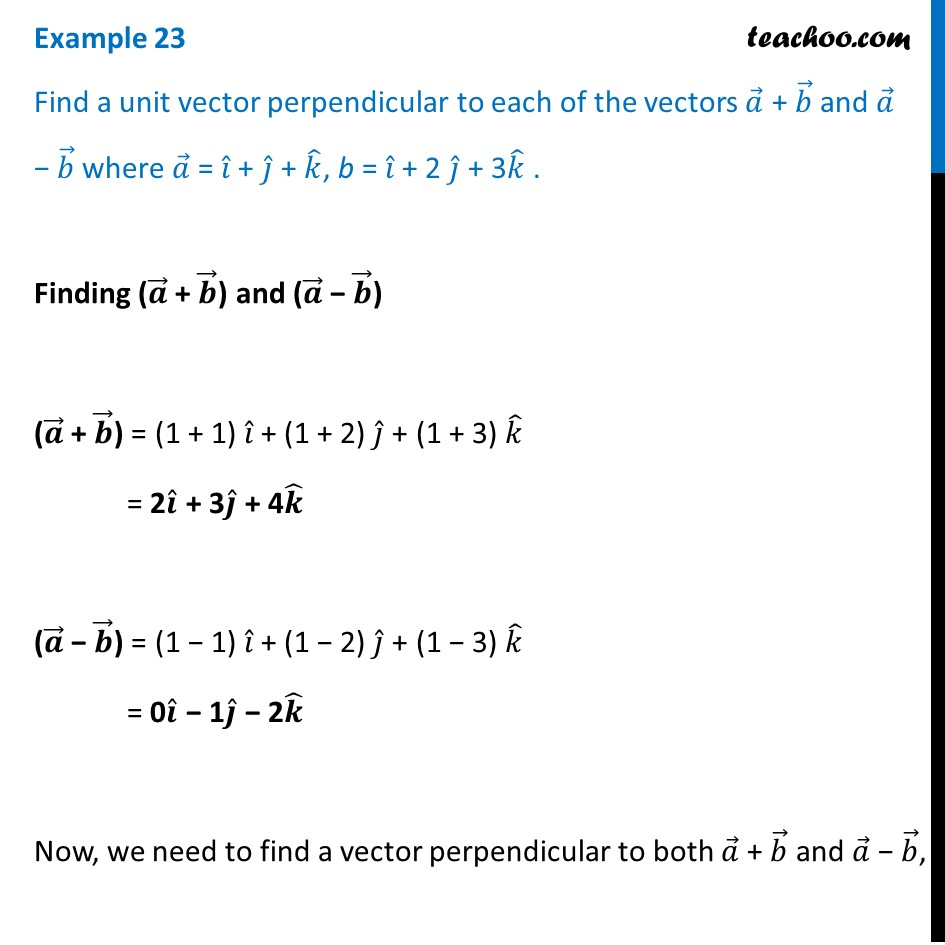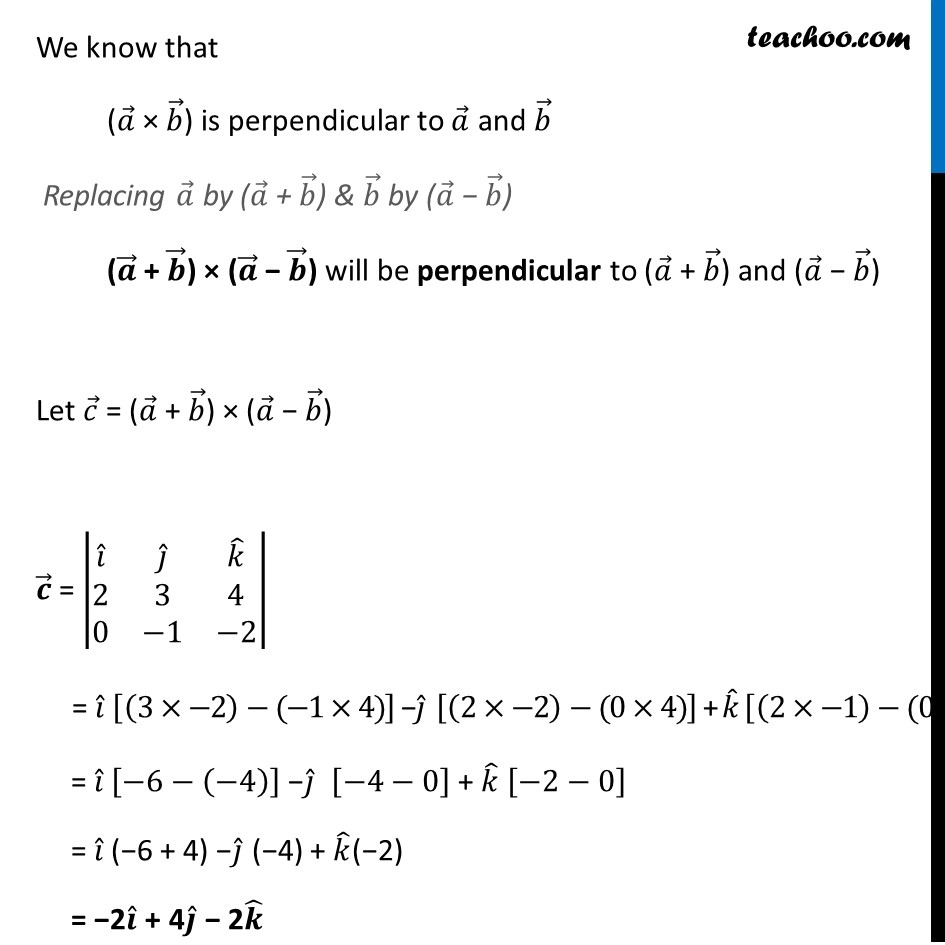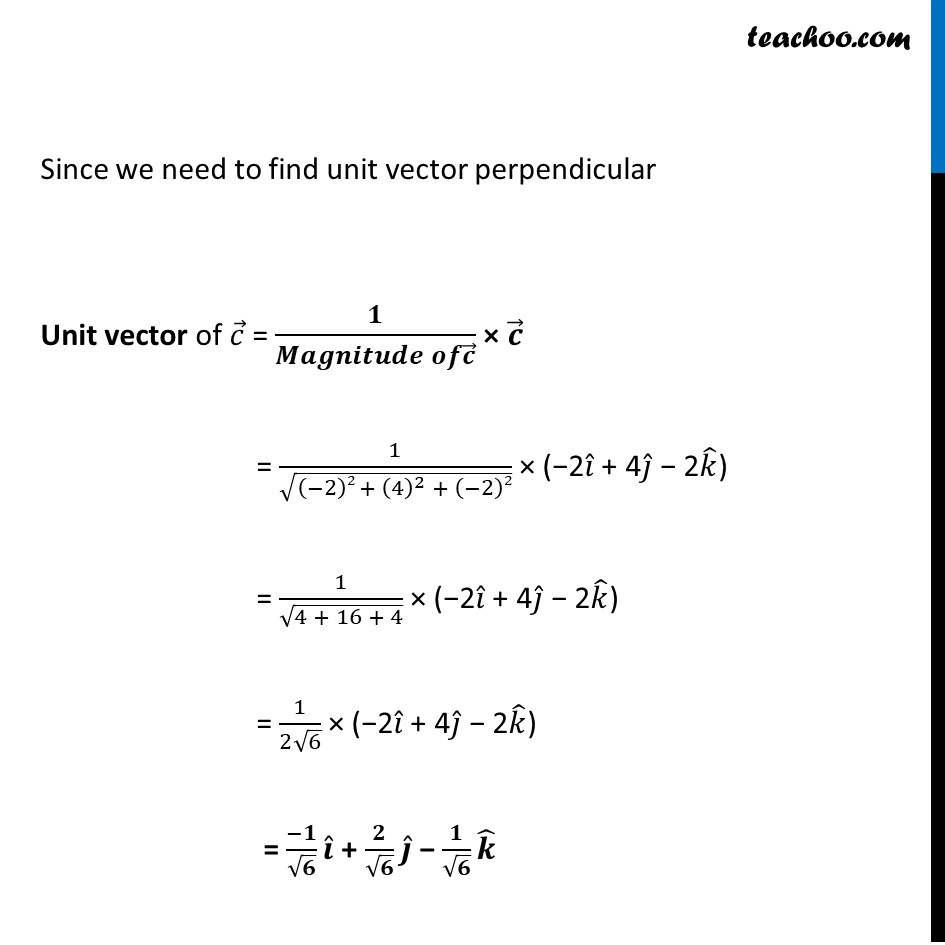This video is only available for Teachoo black users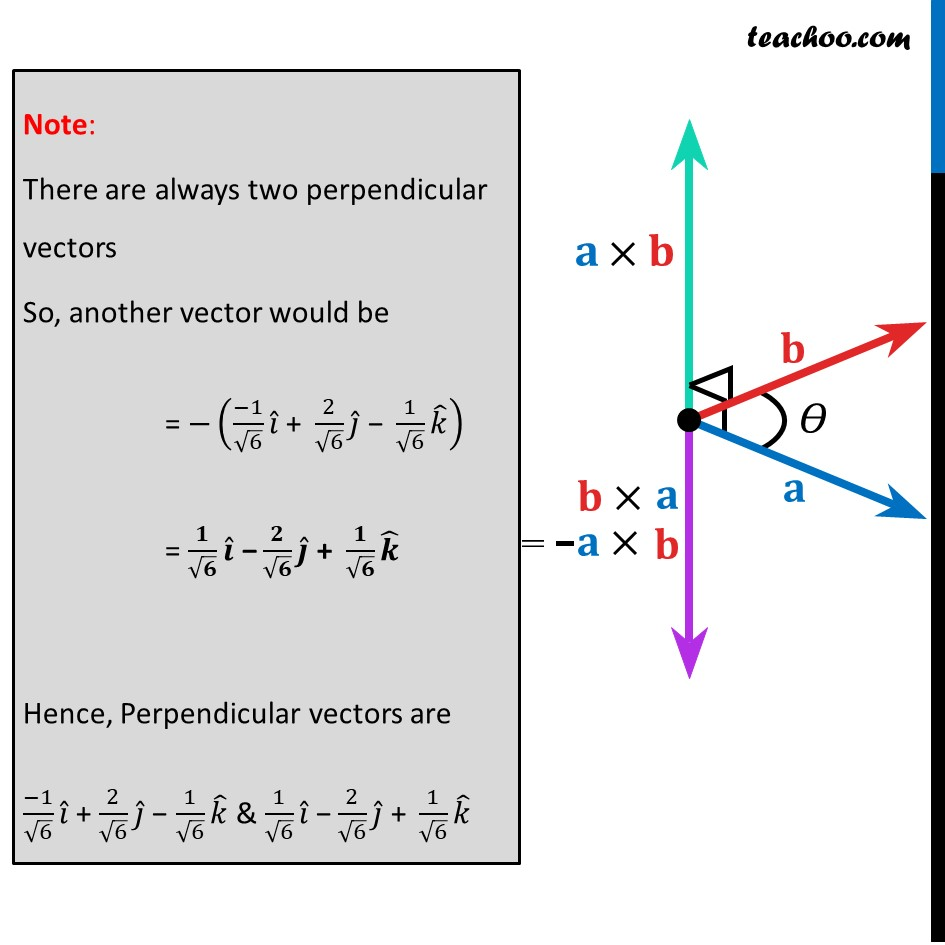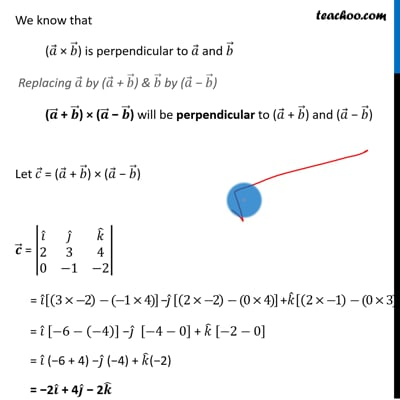This video is only available for Teachoo black users

Introducing your new favourite teacher - Teachoo Black, at only ₹83 per month

### Transcript

Example 23 Find a unit vector perpendicular to each of the vectors 𝑎 ⃗ + 𝑏 ⃗ and 𝑎 ⃗ − 𝑏 ⃗ where 𝑎 ⃗ = 𝑖 ̂ + 𝑗 ̂ + 𝑘 ̂, b = 𝑖 ̂ + 2 𝑗 ̂ + 3𝑘 ̂ . Finding (𝒂 ⃗ + 𝒃 ⃗) and (𝒂 ⃗ − 𝒃 ⃗) (𝒂 ⃗ + 𝒃 ⃗) = (1 + 1) 𝑖 ̂ + (1 + 2) 𝑗 ̂ + (1 + 3) 𝑘 ̂ = 2𝒊 ̂ + 3𝒋 ̂ + 4𝒌 ̂ (𝒂 ⃗ − 𝒃 ⃗) = (1 − 1) 𝑖 ̂ + (1 − 2) 𝑗 ̂ + (1 − 3) 𝑘 ̂ = 0𝒊 ̂ − 1𝒋 ̂ − 2𝒌 ̂ Now, we need to find a vector perpendicular to both 𝑎 ⃗ + 𝑏 ⃗ and 𝑎 ⃗ − 𝑏 ⃗, We know that (𝑎 ⃗ × 𝑏 ⃗) is perpendicular to 𝑎 ⃗ and 𝑏 ⃗ Replacing 𝑎 ⃗ by (𝑎 ⃗ + 𝑏 ⃗) & 𝑏 ⃗ by (𝑎 ⃗ − 𝑏 ⃗) (𝒂 ⃗ + 𝒃 ⃗) × (𝒂 ⃗ − 𝒃 ⃗) will be perpendicular to (𝑎 ⃗ + 𝑏 ⃗) and (𝑎 ⃗ − 𝑏 ⃗) Let 𝑐 ⃗ = (𝑎 ⃗ + 𝑏 ⃗) × (𝑎 ⃗ − 𝑏 ⃗) 𝒄 ⃗ = |■8(𝑖 ̂&𝑗 ̂&𝑘 ̂@2&3&4@0&−1&−2)| = 𝑖 ̂ [(3×−2)−(−1×4)] −𝑗 ̂ [(2×−2)−(0×4)] + 𝑘 ̂ [(2×−1)−(0×3)] = 𝑖 ̂ [−6−(−4)] −𝑗 ̂ [−4−0] + 𝑘 ̂ [−2−0] = 𝑖 ̂ (−6 + 4) −𝑗 ̂ (−4) + 𝑘 ̂(−2) = −2𝒊 ̂ + 4𝒋 ̂ − 2𝒌 ̂ Since we need to find unit vector perpendicular Unit vector of 𝑐 ⃗ = 𝟏/(𝑴𝒂𝒈𝒏𝒊𝒕𝒖𝒅𝒆 𝒐𝒇𝒄 ⃗ ) × 𝒄 ⃗ = 1/√((−2)2 + (4)^2 + (−2)2) × (−2𝑖 ̂ + 4𝑗 ̂ − 2𝑘 ̂) = 1/√(4 + 16 + 4) × (−2𝑖 ̂ + 4𝑗 ̂ − 2𝑘 ̂) = 1/(2√6) × (−2𝑖 ̂ + 4𝑗 ̂ − 2𝑘 ̂) = (−𝟏)/√𝟔 𝒊 ̂ + 𝟐/√𝟔 𝒋 ̂ − 𝟏/√𝟔 𝒌 ̂ Note: There are always two perpendicular vectors So, another vector would be = −((−1)/√6 𝑖 ̂" + " 2/√6 𝑗 ̂" − " 1/√6 𝑘 ̂ ) = 𝟏/√𝟔 𝒊 ̂" −" 𝟐/√𝟔 𝒋 ̂" + " 𝟏/√𝟔 𝒌 ̂ Hence, Perpendicular vectors are (−1)/√6 𝑖 ̂ + 2/√6 𝑗 ̂ − 1/√6 𝑘 ̂ & 1/√6 𝑖 ̂" −" 2/√6 𝑗 ̂" + " 1/√6 𝑘 ̂